# Resources tagged with: Mathematical modelling

Filter by: Content type:
Age range:
Challenge level:

### There are 68 results

Broad Topics > Mathematical Thinking > Mathematical modelling### Engnrich

##### Age 16 to 18 Challenge Level:

engNRICH is the area of the stemNRICH Advanced site devoted to the mathematics underlying the study of engineering### Population Ecology Using Probability

##### Age 16 to 18 Challenge Level:

An advanced mathematical exploration supporting our series of articles on population dynamics for advanced students.### Physnrich

##### Age 14 to 18 Challenge Level:

PhysNRICH is the area of the StemNRICH site devoted to the mathematics underlying the study of physics### Chemnrich

##### Age 14 to 18 Challenge Level:

chemNRICH is the area of the stemNRICH site devoted to the mathematics underlying the study of chemistry, designed to help develop the mathematics required to get the most from your study. . . .### Ramping it Up

##### Age 16 to 18 Challenge Level:

Look at the calculus behind the simple act of a car going over a step.### Predator - Prey Systems

##### Age 16 to 18 Challenge Level:

See how differential equations might be used to make a realistic model of a system containing predators and their prey.### The Not-so-simple Pendulum 1

##### Age 16 to 18 Challenge Level:

See how the motion of the simple pendulum is not-so-simple after all.### Population Dynamics - Part 1

##### Age 16 to 18 Challenge Level:

First in our series of problems on population dynamics for advanced students.### Population Dynamics - Part 3

##### Age 16 to 18 Challenge Level:

Third in our series of problems on population dynamics for advanced students.### Population Dynamics - Part 4

##### Age 16 to 18 Challenge Level:

Fourth in our series of problems on population dynamics for advanced students.### Population Dynamics - Part 5

##### Age 16 to 18 Challenge Level:

Fifth in our series of problems on population dynamics for advanced students.### Population Dynamics - Part 6

##### Age 16 to 18 Challenge Level:

Sixth in our series of problems on population dynamics for advanced students.### Population Dynamics - Part 2

##### Age 16 to 18 Challenge Level:

Second in our series of problems on population dynamics for advanced students.### Population Dynamics Collection

##### Age 16 to 18 Challenge Level:

This is our collection of tasks on the mathematical theme of 'Population Dynamics' for advanced students and those interested in mathematical modelling.### Pdf Stories

##### Age 16 to 18 Challenge Level:

Invent scenarios which would give rise to these probability density functions.### Modelling Assumptions in Mechanics

##### Age 16 to 18

An article demonstrating mathematically how various physical modelling assumptions affect the solution to the seemingly simple problem of the projectile.### Branching Processes and Extinction

##### Age 16 to 18 Challenge Level:

An advanced mathematical exploration supporting our series of articles on population dynamics for advanced students.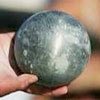### Model Solutions

##### Age 16 to 18 Challenge Level:

How do these modelling assumption affect the solutions?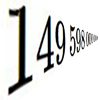### Big and Small Numbers in Physics - Group Task

##### Age 16 to 18 Challenge Level:

Work in groups to try to create the best approximations to these physical quantities.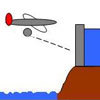### Dam Busters 1

##### Age 16 to 18 Challenge Level:

At what positions and speeds can the bomb be dropped to destroy the dam?### Bionrich

##### Age 14 to 18 Challenge Level:

bioNRICH is the area of the stemNRICH site devoted to the mathematics underlying the study of the biological sciences, designed to help develop the mathematics required to get the most from your. . . .### Population Dynamics

##### Age 16 to 18 Challenge Level:

This problem opens a major sequence of activities on the mathematics of population dynamics for advanced students.##### Age 16 to 18 Challenge Level:

This is the section of stemNRICH devoted to the advanced applied mathematics underlying the study of the sciences at higher levels### The Wrong Stats

##### Age 16 to 18 Challenge Level:

Why MUST these statistical statements probably be at least a little bit wrong?### The Mean Game

##### Age 16 to 18

Edward Wallace based his A Level Statistics Project on The Mean Game. Each picks 2 numbers. The winner is the player who picks a number closest to the mean of all the numbers picked.### Rocking Chairs, Railway Games and Rayboxes

##### Age 5 to 18

In this article for teachers, Alan Parr looks at ways that mathematics teaching and learning can start from the useful and interesting things can we do with the subject, including. . . .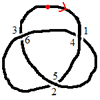### Drawing Doodles and Naming Knots

##### Age 7 to 18

This article for students introduces the idea of naming knots using numbers. You'll need some paper and something to write with handy!### Stonehenge

##### Age 16 to 18 Challenge Level:

Explain why, when moving heavy objects on rollers, the object moves twice as fast as the rollers. Try a similar experiment yourself.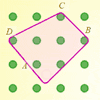### Elastic Maths

##### Age 14 to 18

How do you write a computer program that creates the illusion of stretching elastic bands between pegs of a Geoboard? The answer contains some surprising mathematics.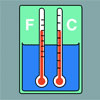### Stemnrich - the Physical World

##### Age 11 to 16 Challenge Level:

PhysNRICH is the area of the StemNRICH site devoted to the mathematics underlying the study of physics### Over-booking

##### Age 16 to 18 Challenge Level:

The probability that a passenger books a flight and does not turn up is 0.05. For an aeroplane with 400 seats how many tickets can be sold so that only 1% of flights are over-booked?### Bird-brained

##### Age 16 to 18 Challenge Level:

How many eggs should a bird lay to maximise the number of chicks that will hatch? An introduction to optimisation.### FA Cup

##### Age 16 to 18 Challenge Level:

In four years 2001 to 2004 Arsenal have been drawn against Chelsea in the FA cup and have beaten Chelsea every time. What was the probability of this? Lots of fractions in the calculations!### Maximum Flow

##### Age 16 to 18 Challenge Level:

Given the graph of a supply network and the maximum capacity for flow in each section find the maximum flow across the network.### Truth Tables and Electronic Circuits

##### Age 11 to 18

Investigate circuits and record your findings in this simple introduction to truth tables and logic.### Guessing the Graph

##### Age 14 to 16 Challenge Level:

Can you suggest a curve to fit some experimental data? Can you work out where the data might have come from?### The Use of Mathematics in Computer Games

##### Age 16 to 18

An account of how mathematics is used in computer games including geometry, vectors, transformations, 3D graphics, graph theory and simulations.### Overarch 2

##### Age 16 to 18 Challenge Level:

Bricks are 20cm long and 10cm high. How high could an arch be built without mortar on a flat horizontal surface, to overhang by 1 metre? How big an overhang is it possible to make like this?### Designing Table Mats

##### Age 11 to 16 Challenge Level:

Formulate and investigate a simple mathematical model for the design of a table mat.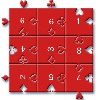### Impuzzable

##### Age 16 to 18

This is about a fiendishly difficult jigsaw and how to solve it using a computer program.### Cushion Ball

##### Age 16 to 18 Challenge Level:

The shortest path between any two points on a snooker table is the straight line between them but what if the ball must bounce off one wall, or 2 walls, or 3 walls?### Time to Evolve 2

##### Age 16 to 18 Challenge Level:

How is the length of time between the birth of an animal and the birth of its great great ... great grandparent distributed?### Spinners

##### Age 16 to 18 Challenge Level:

How do scores on dice and factors of polynomials relate to each other?### Snooker

##### Age 16 to 18 Challenge Level:

A player has probability 0.4 of winning a single game. What is his probability of winning a 'best of 15 games' tournament?### Problem Solving, Using and Applying and Functional Mathematics

##### Age 5 to 18 Challenge Level:

Problem solving is at the heart of the NRICH site. All the problems give learners opportunities to learn, develop or use mathematical concepts and skills. Read here for more information.### An Introduction to Computer Programming and Mathematics

##### Age 16 to 18

This article explains the concepts involved in scientific mathematical computing. It will be very useful and interesting to anyone interested in computer programming or mathematics.### Ball Bearings

##### Age 16 to 18 Challenge Level:

If a is the radius of the axle, b the radius of each ball-bearing, and c the radius of the hub, why does the number of ball bearings n determine the ratio c/a? Find a formula for c/a in terms of n.### In Constantly Passing

##### Age 14 to 16 Challenge Level:

A car is travelling along a dual carriageway at constant speed. Every 3 minutes a bus passes going in the opposite direction, while every 6 minutes a bus passes the car travelling in the same. . . .### Stringing it Out

##### Age 14 to 16 Challenge Level:

Explore the transformations and comment on what you find.### The Legacy

##### Age 14 to 16 Challenge Level:

Your school has been left a million pounds in the will of an ex- pupil. What model of investment and spending would you use in order to ensure the best return on the money?